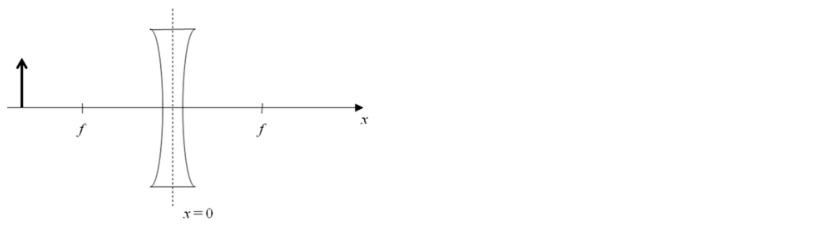# Problem: An object (bold arrow) is located at a distance of 3 f / 2 (i.e. at x = 3 f / 2) in front of a diverging lens as shown in the figure. Note that f is positive, so the focal length of this lens is - f.What is the location x i of the image?a) x i = -3f / 5b) x i = 3f / 2c) x i  = 3f / 2d) x i = f / 2e) x i = f / 5

🤓 Based on our data, we think this question is relevant for Professor Efthimiou's class at UCF.

###### Problem Details

An object (bold arrow) is located at a distance of 3 f / 2 (i.e. at x = 3 f / 2) in front of a diverging lens as shown in the figure. Note that f is positive, so the focal length of this lens is - f.

What is the location x i of the image?

a) x i = -3f / 5

b) x i = 3f / 2

c) x  = 3f / 2

d) x i = f / 2

e) x i = f / 5